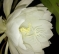Started by 5 years ago6 replieslatest reply 5 years ago125 views

Hi all , my problem is:

A real sequence A,the length is  'N', 'N' is multiple of 32, the pattern is as below:

A : {a(0), a(1), a(2) ..., a(N/2-1), b(0), b(1), b(2),  ... , b(N/2-1)},

the other two sequence is B, C, length is also 'N',as below:

B : {a(0), a(1), a(2) ..., a(N/2-1), 0, ... , 0},

C : {0, ... , 0 , b(0), b(1), b(2) ..., b(N/2-1),},

you can see,sequence A, B, C meet : A = B + C.

the forward FFT of sequence A is known, but sequence A,B,C are all unknown,

I just want to know the forward FFT of sequence B and C,

I know the ordinary method is perform a backward FFT to get sequence A,

then sequence B and C is also known, then perform forward FFT about B, the FFT of C can be get by subtract FFT of B from FFT of A,

but the computaton is too complicated,

Is there a fast method?

[ - ]There is a faster method for smaller N, but it probably isn't fast as you desire.  Any potential savings also depends on the size of N.

If you think of the DFT and inverse DFT as matrix multiplies, then you will realize you only need to do half of the inverse multiplication to recover your "a" sequence.

Doing a forward DFT on your "a" sequence to get the DFT of B will also be half of the multiplication.  As you observed, the DFT of C is obtained by subtracting the DFT of B from the DFT of A.

With this method you never actually calculate your sequence of "b".

As N gets larger, the savings of doing an FFT over a matrix multiply DFT will quickly render this technique useless.

Maybe somebody can improve on this.

Ced
[ - ]Hi Cedron, thank you, N is at least 320, maybe there is no faster algorithm

[ - ]Another reply besides my earlier: Do this on a GPU. You need massively parallel processing. Do this in Python, not C

[ - ]Post deleted by author

[ - ]